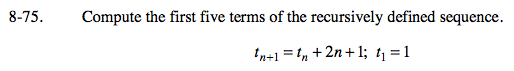### Home > PC > Chapter 8 > Lesson 8.2.1 > Problem8-75

8-75.t = 1
If n = 1, then...
t1+1 = t1 + 2(1) + 1
t2 = 1 + 2(1) + 1
t2 = 4
Now what if n = 2, 3, or 4?